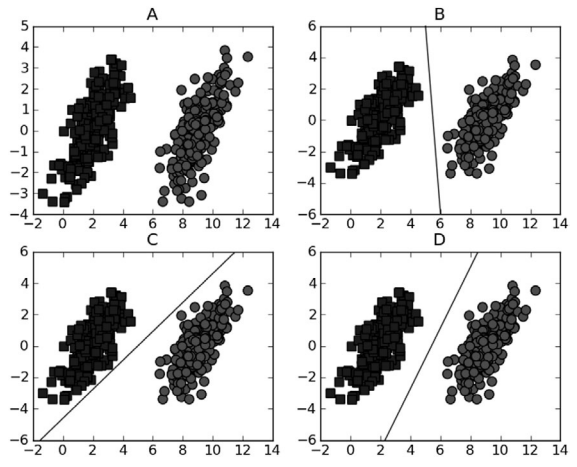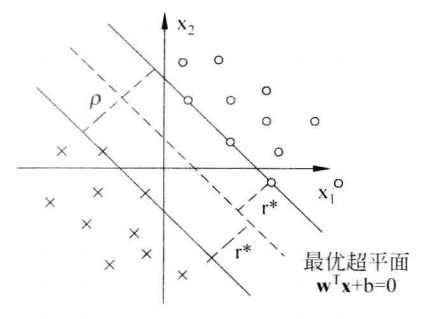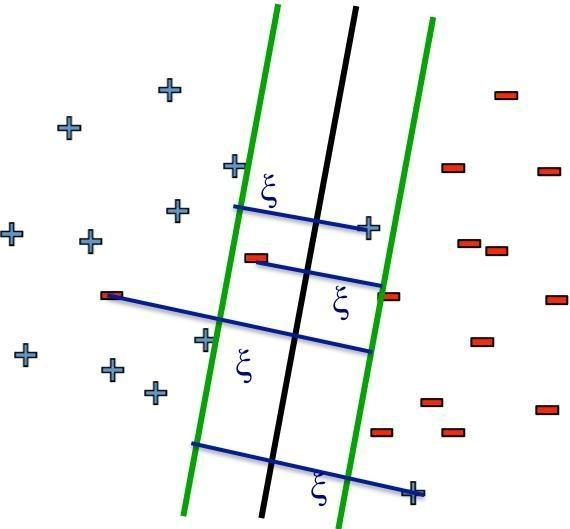SVM# 线性可分SVM

$||w||$ 是向量 $w$ 的内积，即 $||w|| = \sqrt{w_0^2 + w_1^2 + \dots + w_n^2 }$ ，而 $h(x)$ 表示函数间隔：

## 最大化间隔$f(x)$ 是我们需要最小化的目标函数， $g(x)$ 是不等式约束条件， $\alpha$ 是对应的约束系数，也称拉格朗日乘子。为了使得拉格朗日函数得到最优解，我们需要加入能使该函数有最优化解法的KKT条件，或者叫最优化条件。即假设存在一点 $x^*$：

• $L(x^{\star})$ 对 $x^{\star}$ 求导为 $0$
• $\alpha_ig_i(x^*)=0$ 对于所有的 $i = 1,\dots,n$

## 软间隔

• 放宽严格的间隔，构造软间隔
• 运用核函数将数据映射到高维空间，然后在高维空间中寻找超平面进行线性划分。

### $C$ 和 $\xi$• 当 $C$ 很大时，$\xi$ 趋近于0，表示惩罚很大，容忍度很低。这样错分较少，对样本的拟合性较好，但容易过拟合。
• 当 $C$ 很小时，$\xi$ 变大，表示惩罚很小，容忍度高。这样错分较多，对样本的拟合性下降。

## SMO算法

1996年， John Platt发布了一个称为SMO的强大算法，用于训练SVM。 SMO表示序列最小优化（Sequential Minimal Optimization）。 Platt的SMO算法是将大优化问题分解为多个小优化问题来求解，这些小优化问题往往很容易求解，并且对它们进行顺序求解的结果与将它们作为整体来求解的结果是完全一致的。

### 工作原理# 核函数